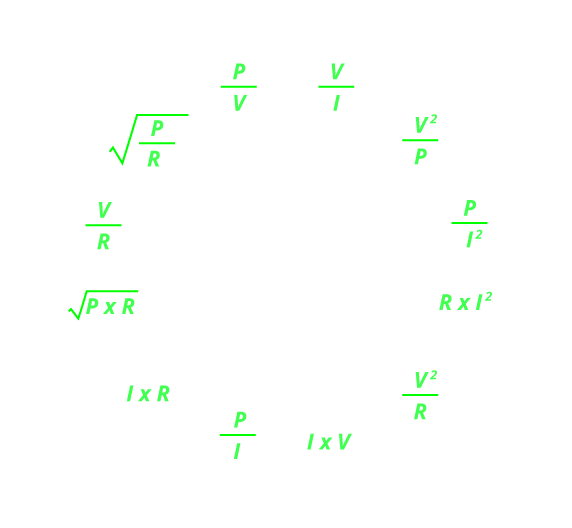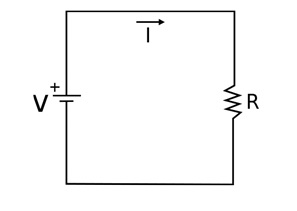(206) 687-4009 | [email protected]

# Ohm's Law Calculator

This ohm’s law calculator is a simple tool to find out the relationships between voltage, current, power and resistance in DC circuits.

Note: Enter any two values
Current(I)
Amps
Voltage(V)
Volts
Power(P)
Watts
Resistance(R)
Ω## Ohm's law:

Ohm’s law shows the relation between voltage and current. It is defined as:

‘The amount of current flowing through a conductor depends directly on potential difference across it.”

the final form of the equation comes out to be:

V = I X R

Where

• V = potential difference or voltage
• I = current
• R = resistance## Power in DC circuits:

Based on ohms law, you can also calculate power. Power is the product of voltage and current, so the equation is as follows:

P = V X I

And we know that,

V = I X R

So,

P =
V2 / R
OR

P = I2 X R

## What are volts?

Volts are the SI unit of potential difference or voltage. it is defined as:

When 1-joule energy is required to move a charge of 1 coulomb it is equal to 1 volt

## What are amps?

Amps are the SI unit of current. It is defined as:

When 1-coulomb charge moves for 1 sec it is equal to 1 amp.

## What are ohms?

Ohms are the SI unit of resistance. it is defined as:

When one-ampere current is subjected to a potential difference of one volt.

## What are watts?

Watts are the SI unit of Power. It is defined as:

When one joule of energy is consumed in one second. It is corresponding to the rate of consumption of energy in an electric circuit where the potential difference is one volt and the current one ampere

## Solved Example:

### When Current and Voltage is known:

Given:

Current = 12 Amps

Voltage = 60 Volts

Required:

Power = ?

Resistance = ?

Solution:

Power = Voltage  Current = 60 x 12

Power = 720 watts

Resistance = Voltage / Current = 60/12

Resistance = 5 ohms

### When Current and Power is known:

Given:

Current = 10 Kilo-Amps

Power = 50 Mega-Watts

Required:

Voltage = ?

Resistance = ?

Solution:

Voltage = Power / Current =
50 x 106/10 * 103
= 5000 Volts = 5 Kilo Volts
Resistance = Power / Current^2 =
50 x 106/(10 x 103)2
Resistance = 0.5 ohms

### When Resistance and current are known:

Given:

Current = 10 Milli-Amps

Resistance = 3 Mega-Ohms

Required:

Voltage = ?

Power = ?

Solution:

Voltage = Resistance  x Current = 10 x 10-3 x 3 x 106

Voltage = 30 Kilo Volts or 30 x 103 Volts

Power = current^2  Resistance =  (10 x 10-3) x 3 x 106

Power = 300 watts or 0.3 Kilo Watts

### UNIT CONVERSIONS:

 1 Kilowatt (KW) 1 x 103 Watts 1 Megawatt (MW) 1 x 106 Watts 1 Gigawatt (GW) 1 x 109 Watts 1 Terawatt (TW) 1 x 1012 Watts

 1 Millivolt (mV) 1 x 10-3 Volts 1 Kilovolt (kV) 1 x 103 Volts

 1 Milliampere (mA) 1 x 10-3 Amperes 1 Kiloampere (kA) 1 x 103 Amperes 1 Microampere (µA) 1 x 10-6 Amperes

 1 Mega Ohm (MΩ) 1 x 106 Ohms 1 Kilo Ohm (kΩ) 1 x 103 Ohms 1 Milli Ohm (mΩ) 1 x 10-3 Ohms